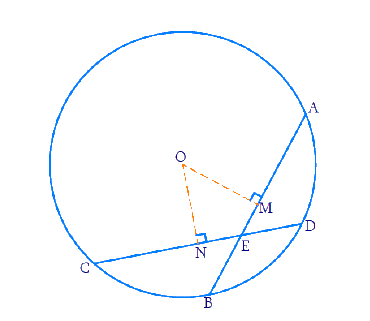In the verge of coronavirus pandemic, we are providing FREE access to our entire Online Curriculum to ensure Learning Doesn't STOP!

# Ex.10.4 Q3 Circles Solution - NCERT Maths Class 9

Go back to  'Ex.10.4'

## Question

If two equal chords of a circle intersect within the circle, prove that the line joining the point of intersection to the center makes equal angles with the chords.

Video Solution
Circles
Ex 10.4 | Question 3

## Text Solution

What is known?

Chords are equal. Chords intersect at a point within the circle.

What is unknown?

Line joining the point of intersection to the centre makes equal angles with the chords.

Reasoning:

Equal chords are equidistant from the centre. Using this and Right angled- Hypotenuse-Side (RHS) criteria and Corresponding parts of congruent triangles (CPCT) we prove the statement.

Steps:Let $${AB}$$ and $${CD}$$ be the $$2$$ equal chords. $${AB = CD.}$$

Let the chords intersect at point $${E}$$. Join $${OE.}$$

Draw perpendiculars from the centre to the chords. Perpendicular bisects the chord $$AB$$ at $$M$$ and $$CD$$ at $$N$$.

To prove: $$\angle {OEN}=\angle {OEM}$$

$${\rm{In}} \;\Delta {OME}\; {\rm{and}} \;\Delta {ONE}$$ \begin{align} \angle {M}&=\angle {N}=90^{\circ}\\ {OE}&={OE}\\ {OM}&={ON} \quad \\\end{align}

( Equal chords are equidistant from the centre.)

By RHS criteria, $$\Delta {OME}$$ and $$\Delta {ONE}$$ are congruent.

$$\text{So by CPCT, }\angle {OEN}=\angle {OEM}$$

Hence proved that line joining the point of intersection of $$2$$ equal chords to the centre makes equal angles with the chords.

Video Solution
Circles
Ex 10.4 | Question 3

Learn from the best math teachers and top your exams

• Live one on one classroom and doubt clearing
• Practice worksheets in and after class for conceptual clarity
• Personalized curriculum to keep up with school